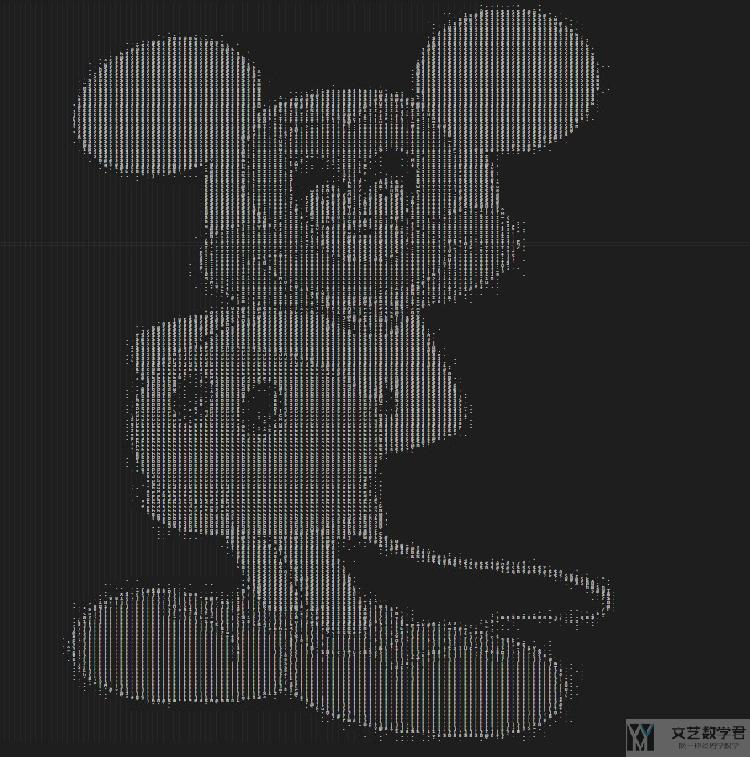# Python生成字符画

• A+

## 简介## 生成的方式和原理

### 源代码

Github仓库链接: Python生成字符画

### 实现原理

• 读入图片, 修改图片大小
• 对每一个像素点进行操作
• 若是彩色, 则转换为灰度
• 将灰度值映射为字符串
• 在转换为字符串的时候, 因为像素块是正方形的, 字符串是长方形的, 即一般的字母都是长比宽大, 所以我们在实际处理的时候, 会在每个字符后面加上一个空格, 来缓解一下最终输出时候长和宽不对等的情况. 这个也就是对应代码中的, txt += '  '.
• 将最终的结果进行保存

### 代码实现

1. from PIL import Image
2. import fire
3. ascii_char = list("\$@B%8&WM#*oahkbdpqwmZO0QLCJUYXzcvunxrjft/\|()1{}[]?-_+~<>i!lI;:,\"^`'. ")
4. # 将256灰度映射到70个字符上-即输入一个像素点,输出一个符号
5. def get_char(r,g,b,alpha = 256):
6.     if alpha == 0:
7.         return ' '
8.     length = len(ascii_char)
9.     # 将彩色转换为灰度
10.     gray = int(0.2126 * r + 0.7152 * g + 0.0722 * b)
11.     unit = (256.0 + 1)/length
12.     return ascii_char[int(gray/unit)]
13. def image_process(input_file, output_file='res.txt', pic_height=100, pic_weight=100):
14.     # 加载图片
15.     im = Image.open(input_file)
16.     # 调整图片大小
17.     im = im.resize((pic_weight,pic_height), Image.NEAREST)
18.     # 保存缩小后的图片
19.     # im.save('temp.jpg')
20.     txt = ""
21.     # 对每个像素点进行操作
22.     for i in range(pic_height):
23.         for j in range(pic_weight):
24.             txt += get_char(*im.getpixel((j,i)))
25.             txt += '  '
26.         txt += '\n'
27.     print(txt)
28.     #字符画输出到文件
29.     if output_file:
30.         with open(output_file,'w') as f:
31.             f.write(txt)
32.     else:
33.         with open("output.txt",'w') as f:
34.             f.write(txt)
35. if __name__ == '__main__':
36.     """
37.     input_file: 图像的名称
38.     output_file: 保存文件的名称
39.     """
40.     fire.Fire(image_process)

1. python char_pic.py ../char_pic/62.jpg## 一些其他的• 微信公众号
• 关注微信公众号
•• QQ群
• 我们的QQ群号
•